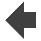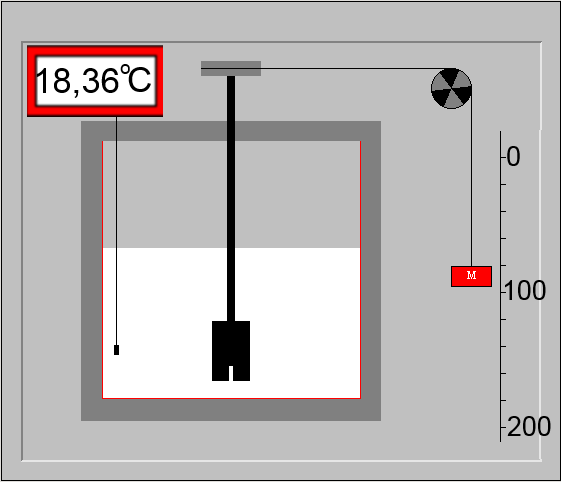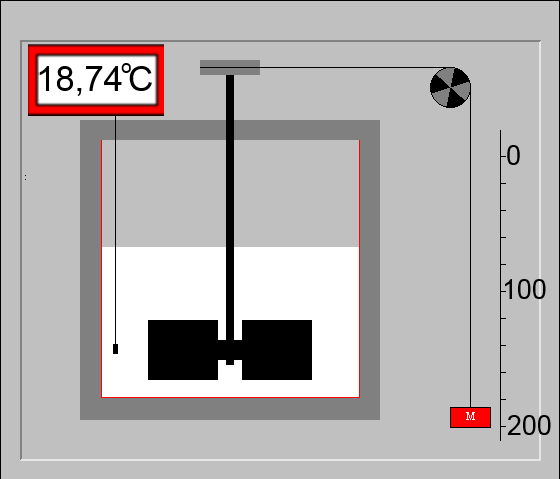Search:

# Heat and work

## Joule's experiment

Click on the arrow on top !Author: →   Université du MansIn this experiment, $m\; g$ of water, placed in a calorimeter, are heated by rotating fans moved by a mass $M$ falling down from a height $h$. The full conversion of work to heat is shown by the temperature rise $\Delta\;T$ of the liquid: Amount of heat received by the water of the calorimeter = $c_{water}\cdot m_{water}\cdot \Delta T$ Work done by the weight $P$ of mass $M$ = $P\cdot g \cdot h$ $=$ $M \cdot g \cdot h$

## "Equivalence" between work and heat

Joule supposed that work is entirely transformed into heat: $M\cdot g\cdot h$ $=$ $m_{water}\cdot c_{water}\cdot \Delta T$ Knowing that in this experiment: $M$ $=$ $24 kg$ $h$ $=$ $2 m$ and remembering that $c_{water}$ $=$ $1\frac{cal}{gK}$ $m_{water}$ $=$ $150 g$ $g$ $=$ $9.81 \frac{N}{kg}$, we have: $24kg\cdot 9.81\frac{N}{kg}\cdot 2m$ $=$ $150g\cdot 1\frac{cal}{gK}\cdot (18.74-18)K$ $470.9\; J$ $=$ $111 cal$ $1cal$ $\approx$ $4.2\; J$

## Energy: heat or work

Calorie and Joule are two "equivalent" units of what is called energy. Here is the correct conversion of these units:

$1\; cal = 4.184\; J$ $1\; J = 0.24\; cal$